2019/09/06

# 常见的RNN结构

## N vs N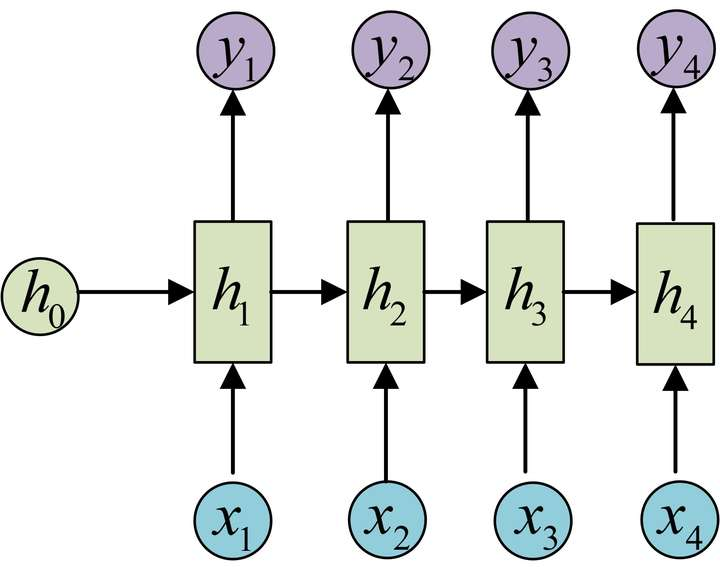• 说明
• 圆圈或方块表示的是向量
• 箭头表示对某个向量做一次变换，具体地有$h_i=f\left( Ux_i+Wh_{i-1}+b \right)$,$y_i=soft\max \left( Vh_i+c \right)$ 其中$i=1,2,3,4$
• 上图中每一步的权重$U,W,V$和偏置$b,c$参数都是一样的，即每一步的参数是共享的
• 应用
• char rnn 即输入为字符，输出为下一个字符的概率。其可用来生成文章、诗歌、代码等。

## N vs 1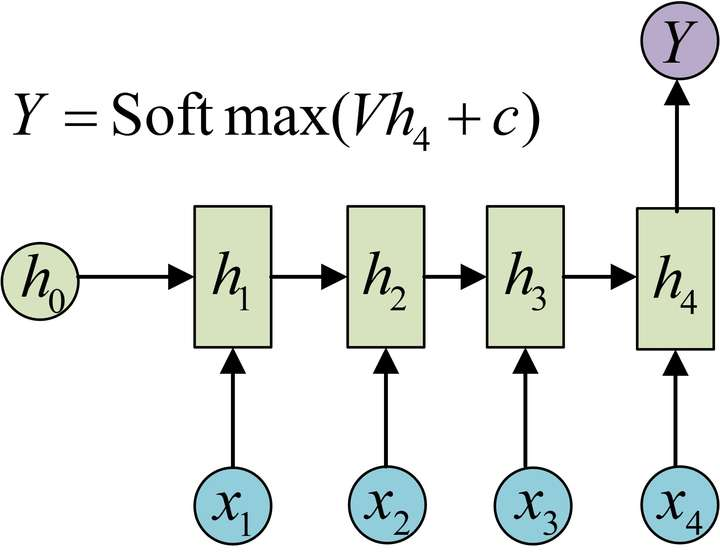• 应用
• 上述结构经常用来处理序列分类问题。

## 1 VS N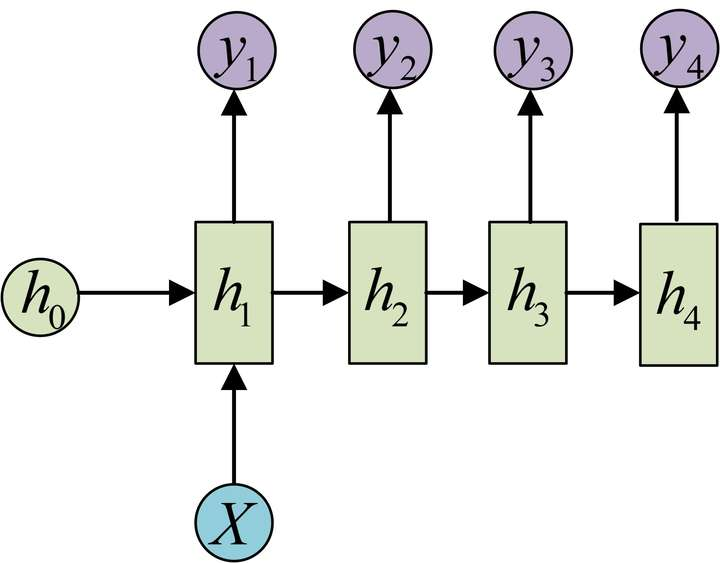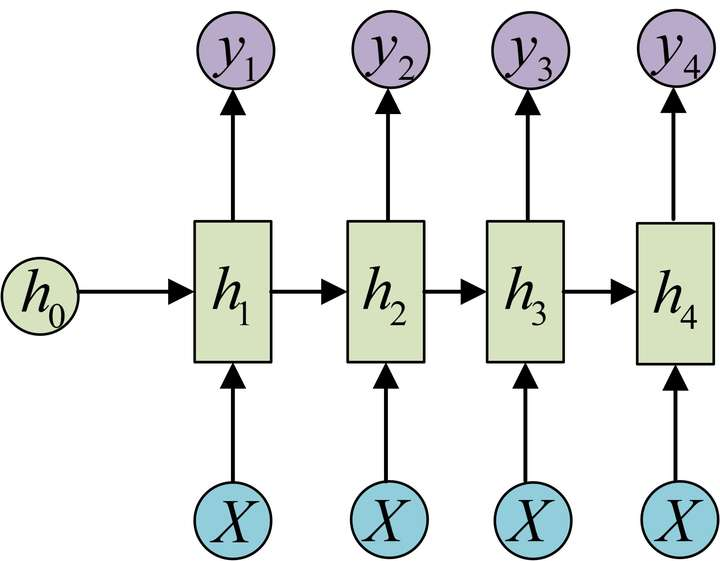• 应用
• 由图像生成文字
• 由类别生成语音或音乐

## N vs M (Encoder-Decoder/seq2seq)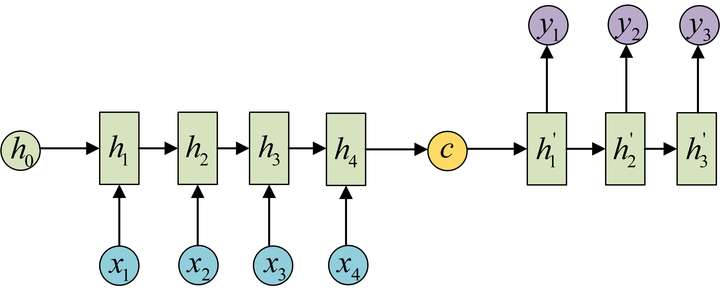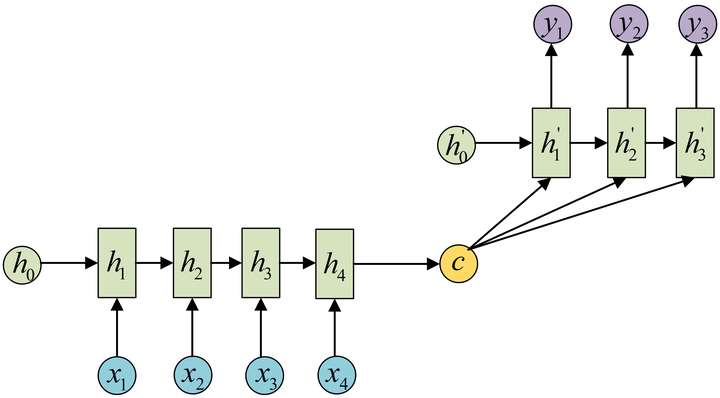• 说明
• 上下文向量$c$可有多种表示形式。最简单的方法可把Encoder的最后一个隐状态赋值给$c$，还可对最后一个隐状态$h_4$做一个变换，也可对所有的隐状态做变换得到。
• 应用
• 机器翻译
• 文本摘要
• 阅读理解。将输入的文章和问题分别编码，解码后得到问题的答案
• 语音识别。输入的是语音信号序列，输出的是文字序列

# tensorflow中的RNN

## 单步RNN: RNNCell

RNNCell是tensorflow中实现RNN的基本单元，每个RNNCell都有一个call方法，使用方式如下

(output, next_state) = call(input, state)


• state_size (隐层大小)
• output_size (输出大小) 两个属性值大小相同，都等于num_units

import tensorflow as tf
import numpy as np

cell = tf.nn.rnn_cell.BasicRNNCell(num_units=128) # state_size = 128
print(cell.state_size) # 128

inputs = tf.placeholder(np.float32, shape=(32, 100)) # 32 是 batch_size
h0 = cell.zero_state(32, np.float32) # 通过zero_state得到一个全0的初始状态，形状为(batch_size, state_size)
output, h1 = cell.__call__(inputs, h0) # 调用call函数

print(h1.shape) # (32, 128)
print(output.shape) # (32, 128)


BasicLSTMCell有两个隐状态$h,c$，对应的隐层是一个tuple，每个隐层的形状都是(batch_size,state_size)

import tensorflow as tf
import numpy as np

cell = tf.nn.rnn_cell.BasicLSTMCell(num_units=128) # state_size = 128
print(cell.state_size) # LSTMStateTuple(c=128, h=128)
print(cell.state_size.h) # 128

inputs = tf.placeholder(np.float32, shape=(32, 100)) # 32 是 batch_size
h0 = cell.zero_state(32, np.float32) # 通过zero_state得到一个全0的初始状态，形状为(batch_size, state_size)
print(h0) # LSTMStateTuple(c=<tf.Tensor 'BasicLSTMCellZeroState/zeros:0' shape=(32, 128) dtype=float32>, h=<tf.Tensor 'BasicLSTMCellZeroState/zeros_1:0' shape=(32, 128) dtype=float32>)
output, h1 = cell.__call__(inputs, h0) #调用call函数

print(h1) # LSTMStateTuple(c=<tf.Tensor 'basic_lstm_cell/Add_1:0' shape=(32, 128) dtype=float32>, h=<tf.Tensor 'basic_lstm_cell/Mul_2:0' shape=(32, 128) dtype=float32>)
print(output.shape) # (32, 128)



## 堆叠RNNCell: MultiRNNCell

import tensorflow as tf
import numpy as np

# 每调用一次这个函数就返回一个BasicRNNCell
def get_a_cell():
return tf.nn.rnn_cell.BasicRNNCell(num_units=128)
# 用tf.nn.rnn_cell MultiRNNCell创建3层RNN
cell = tf.nn.rnn_cell.MultiRNNCell([get_a_cell() for _ in range(3)]) # 3层RNN
# 得到的cell实际也是RNNCell的子类
# 它的state_size是(128, 128, 128)
# (128, 128, 128)并不是128x128x128的意思
# 而是表示共有3个隐层状态，每个隐层状态的大小为128
print(cell.state_size) # (128, 128, 128)
# 使用对应的call函数
inputs = tf.placeholder(np.float32, shape=(32, 100)) # 32 是 batch_size
h0 = cell.zero_state(32, np.float32) # 通过zero_state得到一个全0的初始状态
print(h0) # tuple中含有3个32x128的向量
output, h1 = cell.call(inputs, h0)
print(h1) # tuple中含有3个32x128的向量
print(output.shape) # (32, 128)


## 一次执行多步: tf.nn.dynamic_rnn

MultiRNNCell与RNNCell有一个明显的问题：调用call方法进行运算时，一次只能执行一步。比如使用$x_1,h_0$得到$h_1$，通过$x_2,h_1$得到$h_2$等。如果一个序列的长度为10，则需调用10次call函数，比较麻烦。而通过tf.nn.dynamic_rnn可实现一次执行多步。即通过$\left\{h_0,x_1,x_2,\cdots,x_n\right\}$直接得到$\left\{h_1,h_2,\cdots,h_n\right\}$

dynamic_rnn模型不像MultiRNNCell与RNNCell那样需要显式地调用call方法进行计算，只需在原有单步Cell的基础上调用如下方法即可实现RNN的计算。

# inputs: shape = (batch_size, num_steps, input_size)
# cell: RNNCell
# initial_state: shape = (batch_size, cell.state_size)。初始状态。一般可以取零矩阵
outputs, state = tf.nn.dynamic_rnn(cell, inputs, initial_state=initial_state)


Char RNN